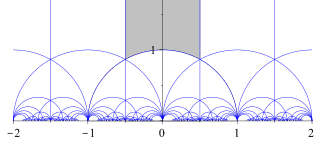# Modular Forms-Fundamental Domain

• I
Apologies if this is a stupid question, but is the fundamental domain unique?

And what exactly is the definition of a cusp- a quick google tells me it is 'where two curves meet', so looking at the fundamental domain,I would say ##\omega=\exp^{\frac{2\pi i}{ 3}} ## and ##\omega*## are?

thanks

.Scott
Homework Helper
https://en.wikipedia.org/wiki/Cusp_(singularity)

For modular forms, the cusps would be all those points along the X axis.mathwonk